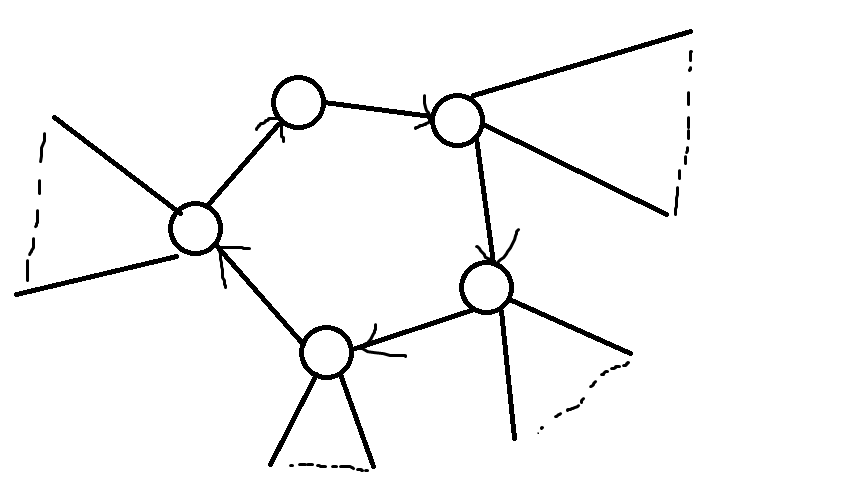BZOJ1040

### 题解• $G[i]$环上第一个点可以选，第$i$个点选时的最大权值和。

• $G[i]$环上第一个点可以选，第$i$个点不选时的最大权值和。

• $G[i]$环上第一个点不可以选，第$i$个点选时的最大权值和。

• $G[i]$环上第一个点不可以选，第$i$个点不选时的最大权值和。